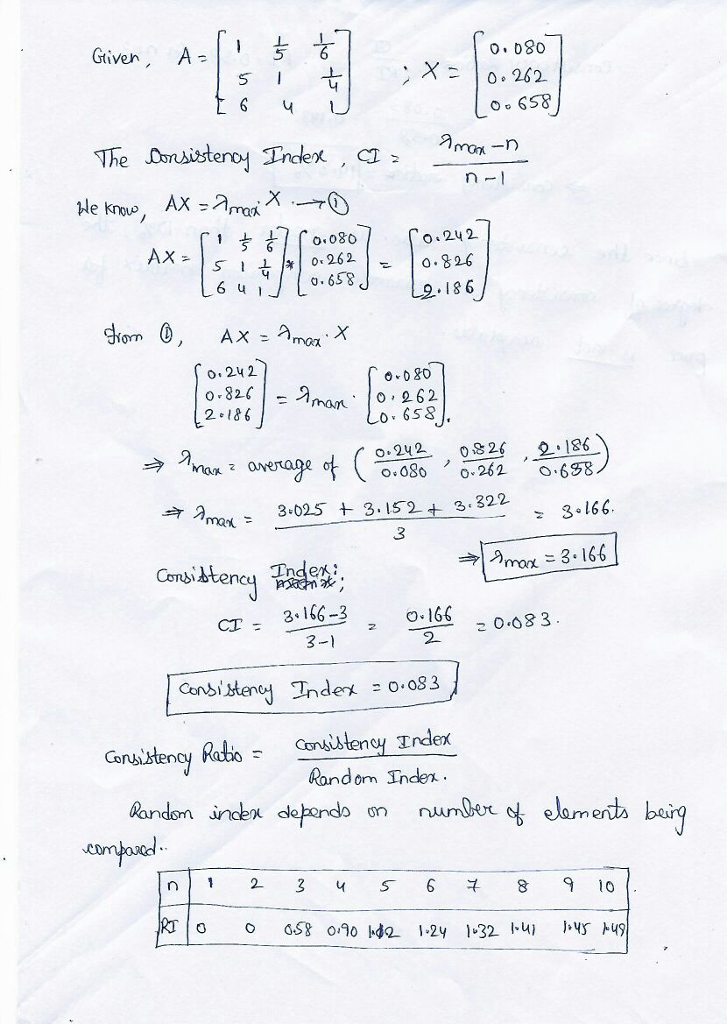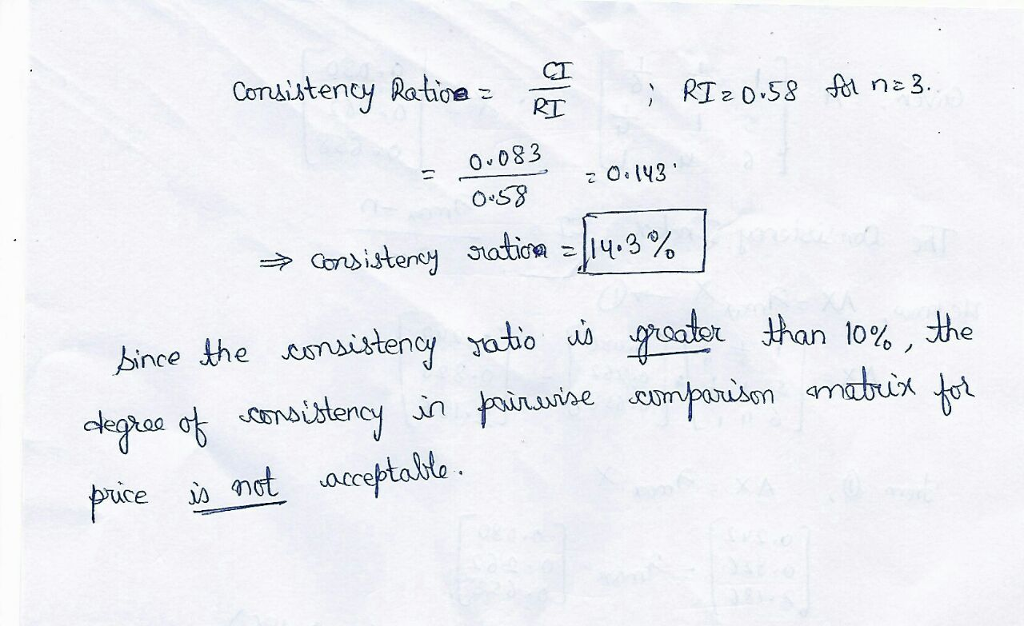# Question & Answer: Problem 14-15 (Algorithmic) Use the pairwise comparison matrix for the pric…..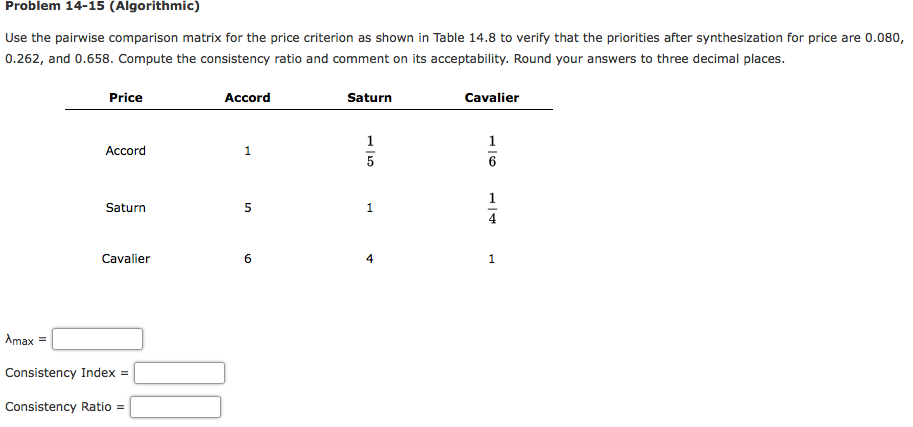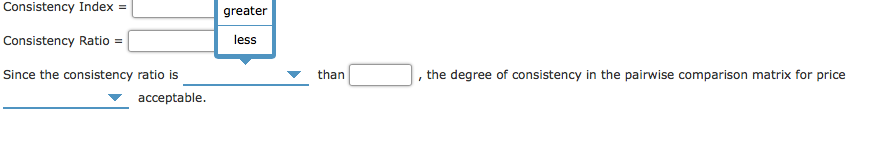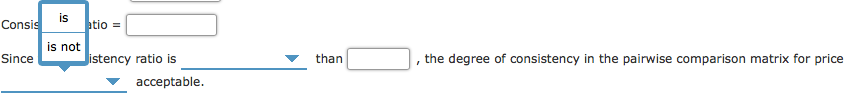Problem 14-15 (Algorithmic) Use the pairwise comparison matrix for the price criterion as shown in Table 14.8 to verify that the priorities after synthesization for price are 0.080, 0.262, and 0.658. Compute the consistency ratio and comment on its acceptability. Round your answers to three decimal places. Price Accord Saturn Cavalier Accord Saturn Cavalier 4 Amax = Consistency Index = Consistency Ratio =